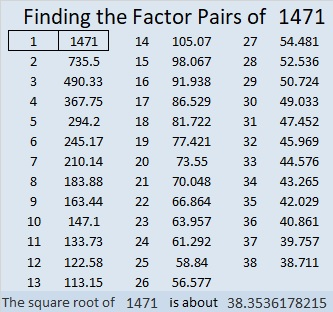# 1471 and Level 3

Contents

### Today’s Puzzle:

There is only one factor that will work with the clue in the top row of this level 3 puzzle. Find it, then work your way down row by row until you have found all the factors. There is only one solution.### Factors of 147

• 1471 is a prime number.
• Prime factorization: 1471 is prime.
• 1471 has no exponents greater than 1 in its prime factorization, so √1471 cannot be simplified.
• The exponent in the prime factorization is 1. Adding one to that exponent we get (1 + 1) = 2. Therefore 1471 has exactly 2 factors.
• The factors of 1471 are outlined with their factor pair partners in the graphic below.How do we know that 1471 is a prime number? If 1471 were not a prime number, then it would be divisible by at least one prime number less than or equal to √1471. Since 1471 cannot be divided evenly by 2, 3, 5, 7, 11, 13, 17, 19, 23, 29, 31, or 37, we know that 1471 is a prime number.

### Another Fact about the Number 1471:

1471 is the 21st centered heptagonal number because
(7(21²)-7(21)+2)/2 = 1471.

This site uses Akismet to reduce spam. Learn how your comment data is processed.